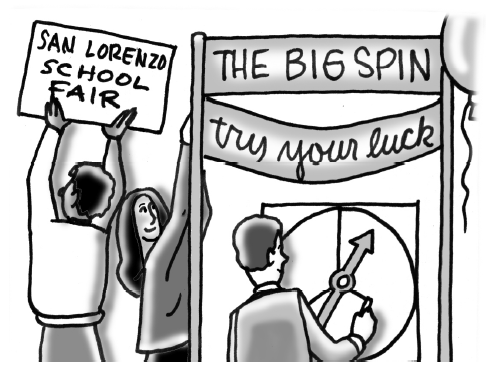### Home > INT2 > Chapter 8 > Lesson 8.4.2 > Problem8-97

8-97.For a school fair, Donny is going to design a spinner with red, white, and blue regions. Since he has a certain proportion of three types of prizes, he wants $P\text{(red)}=40\%$ and $P\text{(white)}=10\%$.

1. If the spinner only has red, white, and blue regions, then what is $P\text{(blue)}$? Explain how you know.

What must the probabilities add up to?

$50\%$

2. What are the central angles of this spinner if it has only three sections? Draw a sketch of the spinner. Be sure to label the regions accurately.

Convert the probability from a percent to a decimal and multiply that by $360^\circ$ to get the central angle.

red $= 144^\circ$; white $= 36^\circ$; blue $= 180^\circ$

1. Is there a different spinner that has the same probabilities? If so, sketch another spinner that has the same probabilities. If not, explain why there is no other spinner with the same probabilities.

Think about a spinner with more than three regions but still only red, white, and blue.